## Arithmetic operators

Here are the most common arithmetic operators*, / and % will be performed before + or - in any expression. Brackets can be used to force a different order of evaluation to this. Where division is performed between two integers, the result will be an integer, with remainder discarded. Modulo reduction is only meaningful between integers. If a program is ever required to divide a number by zero, this will cause an error, usually causing the program to crash.

Here are some arithmetic expressions used within assignment statements.

```
velocity = distance / time;

force = mass * acceleration;

count = count + 1;```

C has some operators which allow abbreviation of certain types of arithmetic assignment statements.These operations are usually very efficient. They can be combined with another expression.Versions where the operator occurs before the variable name change the value of the variable before evaluating the expression, so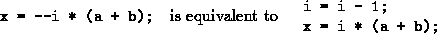These can cause confusion if you try to do too many things on one command line. You are recommended to restrict your use of ++ and - to ensure that your programs stay readable.

Another shorthand notation is listed below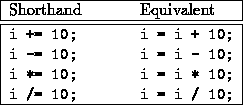These are simple to read and use.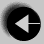Go to Assignment Statement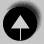Go to Index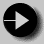Go to Type conversion